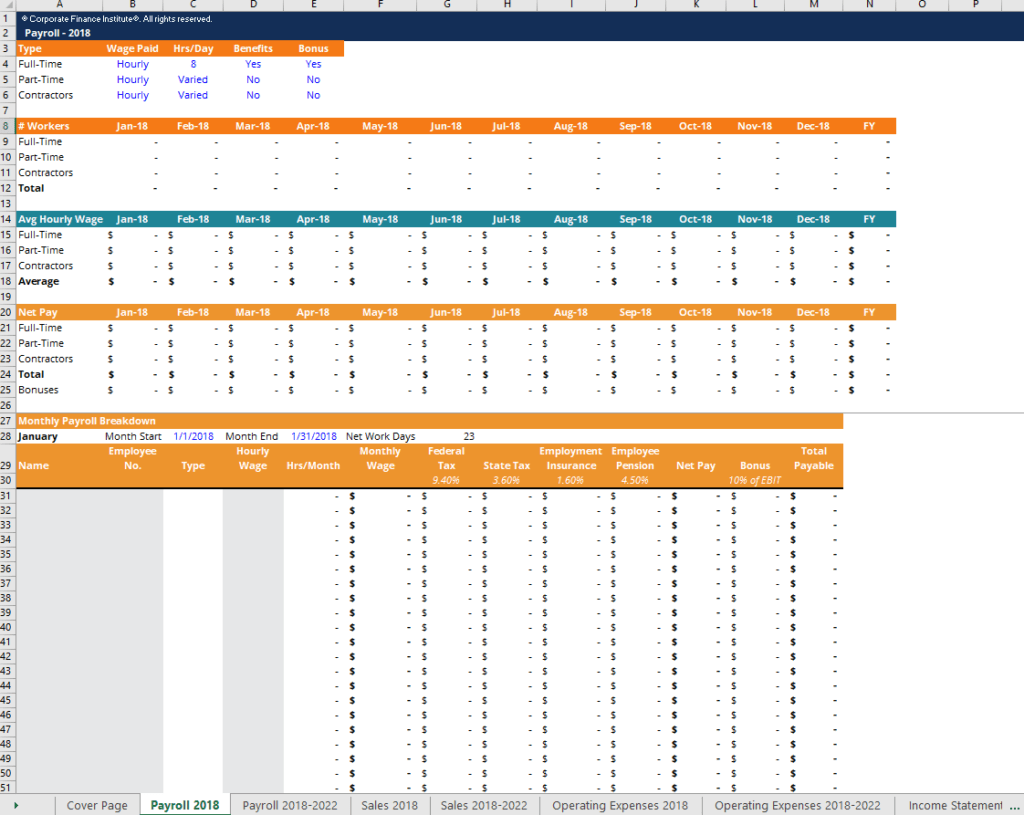# Financial Projection Template

Building up forecast from payroll schedules, operating expenses schedules and sales forecast to the three financial statements

## Financial Projection Template

This financial projection template will help you forecast future revenues and expenses by building up from payroll schedules, operating expenses schedules and sales forecast to the three financial statements.

Below is a screenshot of the financial projection template:### Components of a financial projection template

This financial projection template contains the following sections:

##### #1 Payroll (current year)

In the payroll (current year) worksheet, you will input the payroll expenses for each of the full-time employees, part-time employees, and contractors. The model helps you break down the salary, taxes, employee insurance, pension and employee bonus expenses so you can easily track the total amount for each of the items. These individual monthly payrolls then roll up to the summary tables, which automatically calculate the average hourly wage and net pay for each month (all expenses except bonuses) by staff type.

##### #2 Payroll (forecast)

In the payroll (forecast) worksheet, you will put your own assumptions for the growth rate of the number of workers for the period of forecast. These are the only manual inputs required for the model. Once the assumptions are filled in, the pre-entered formulas will generate the payroll forecast for the rest of the period and calculate average hourly wages by staff type. You’ll also be able to estimate the total amount of taxes, employee insurance, and pension expenses for each of the years.

##### #3 Sales (current year)

In the sales (current year) worksheet, you’ll input the per unit sales price, number of units sold, and per unit cost of goods sold for each product line under the “sales breakdown” section. The model will automatically calculate the monthly revenue, COGS and gross margin for each product line, which are linked to the summary tables at the top of the worksheet. You’ll be able to quickly understand the sales and margin for each product for the current year.

##### #4 Sales (forecast)

In the sales (current year) worksheet, assumptions on sales growth rate are entered for the forecast period to generate the predicted revenue, COGS and gross margin for the following years.

##### #5 Operating expenses (current year)

The operating expenses (current year) worksheet is for you to enter the actual operating expenses for the current year. These will help build up the forecast for operating expenses and the income statement.

##### #6 Operating expenses (forecast)

In this operating expenses (forecast) worksheet, the operating expenses forecast for the next few years will be calculated using the assumptions for each of the expenses items.

##### #7 Income statement (current & forecast)

The income statements for current year and forecast period are built up by linking to values in the sales worksheets and operating expenses worksheets.

##### #8 Balance sheet (current year)

This balance sheet worksheet consists of two main sections: balance sheet and supporting schedules. Balance sheet items such as accounts receivable, inventory, accounts payable and retained earnings will be manually input, while items such as cash, property and equipment, and long-term debt will be linked to other parts of this financial projection template. For example, the debt & interest schedule under the supporting schedules section will help you compute the amount of debt closing and interest expenses, which will then be linked back up to the balance sheet as long-term debt and to the income statement as interest expense.

##### #9 Balance sheet (forecast)

This balance sheet forecast worksheet is built up by taking the current year balance sheet and calculating the following years’ values using assumptions such as accounts receivable days, inventory days, accounts payable days and capital expenditures.

##### #10 Cash flow statement (current year & forecast)

The cash flow statements for the current year and forecast period are constructed using figures calculated in the income statement, balance sheet and supporting schedules. The closing cash balance for each month will be linked back to the balance sheet, shown as cash under current assets.

##### #11 Financial ratio analysis

The final section of this financial projection template is the financial ratio analysis. This worksheet will show you the list of all commonly used financial ratios including profitability ratios, efficiency ratios, liquidity ratios, leverage ratios and coverage ratios, which are calculated using all the worksheets previously built. These ratios will allow you to understand the financial stability of the company and its expected performance in the following years.

### More Free Templates

For more resources, check out our business templates library to download numerous free Excel modeling, PowerPoint presentation, and Word document templates.

• Excel Modeling Templates
• PowerPoint Presentation Templates
• Transaction Document Templates

### Financial Analyst Certification

Become a certified Financial Modeling and Valuation Analyst (FMVA)® by completing CFI’s online financial modeling classes and training program!